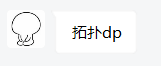# CSP-J/S 2:

## 比赛日:

### CSP-J:

T1 神(s)怖(b)题，当场切掉。

T2 一看，MD普及T2考 Treap？？！当场自闭，然后看到 $a_i\leq 600$，啊这。赛后 xjq爷 说用对顶堆，我觉得有理。

T4暴力打完，回到T3写暴力，暴力都调了好久，我爱死字符串了。

$f_{i,j}=\left\{\begin{matrix}\min_k\left\{f_{k,j-1}+\sum_{l=i}^{k}a_{l,j}\right\}\quad(i\leq k) \\ \min_k\left\{f_{k,j-1}+\sum_{l=k}^{i}a_{l,j}\right\}\quad(i> k) \end{matrix}\right.$

### CSP-S:

T2 感觉可以做，敲完，感觉可以A。

T3，分析一波，不太可能是数据结构，写暴力吧。posted @ 2020-11-13 21:15  Jayun  阅读(47)  评论(0编辑  收藏